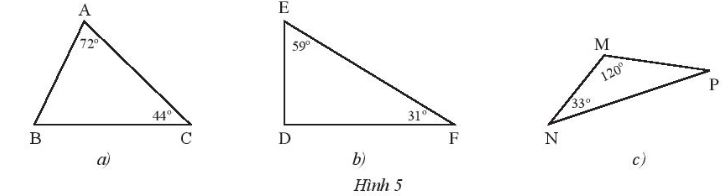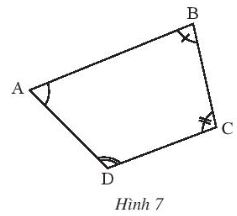## Problem Solving Lesson 1: Angles and sides of a triangle (C8 Math 7 Horizons) – Math Book

Solving Exercise 1: Angles and sides of a triangle (C8 Math 7 Horizons)
————

### Solution 1 page 46 Math textbook 7 Creative horizon volume 2 – CTST

Find the measures of the unknown angles of the triangles in Figure 5.## Detailed instructions for solving Lesson 1

Solution method

Use the theorem about the sum of the angles in a triangle

Detailed explanation

a) According to the theorem about the sum of the measures of the three angles in a triangle, we have:

$$\begin{array}{l}\widehat A + \widehat B + \widehat C = {180^o}\\ \Rightarrow \widehat B = {180^o} – \widehat A – \widehat C = { 180^o} – {72^o} – {44^o} = {64^o}\end{array}$$

b) According to the theorem about the sum of the measures of 3 angles in a triangle, we have:

$$\begin{array}{l}\widehat D + \widehat E + \widehat F = {180^o}\\ \Rightarrow \widehat D = {180^o} – \widehat E – \widehat F = { 180^o} – {59^o} – {31^o} = {90^o}\end{array}$$

c) According to the theorem about the sum of the measures of 3 angles in a triangle, we have:

$$\begin{array}{l}\widehat M + \widehat N + \widehat P = {180^o}\\ \Rightarrow \widehat P = {180^o} – \widehat M – \widehat N = { 180^o} – {120^o} – {33^o} = {27^o}\end{array}$$

–>

— *****

### Solution 2 page 47 Math textbook 7 Creative horizon volume 2 – CTST

Calculate the measure x of the angle in Figure 6.## Detailed instructions for solving Lesson 2

Solution method

Use the sum of the three angles theorem in a triangle

Detailed explanation

a) Call MP perpendicular to NL (P belongs to NL)

Since triangle MNL is right-angled at M, we have

$$\Rightarrow \widehat L = {90^o} – \widehat N = {28^o}$$

Considering triangle MPL, right angled at P, we have:

$$\Rightarrow \widehat x = {90^o} – \widehat L = {90^o} – {28^o} = {62^o}$$

b) Let QF be perpendicular to RP (F belongs to RP)

Since triangle RQP is right-angled at Q, we have:

$$\Rightarrow \widehat R + \widehat P = {90^o} \Rightarrow \widehat P = {90^o} – {52^o} = {38^o}$$

Since the triangle QFP is right angled at F $$\Rightarrow \widehat x + \widehat P = {90^o} \Rightarrow \widehat x = {90^o} – {38^o} = {52^o}$$

–>

— *****

Divide quadrilateral ABCD in Figure 7 into two triangles to find the sum of the measures of the four angles $$\widehat A$$,$$\widehat B$$,$$\widehat C$$,$$\widehat D\ ).## Detailed instructions for solving Lesson 3 Solution method We divide the quadrilateral into 2 triangles Apply the sum of the three angles in a triangle Detailed explanation We divide quadrilateral ABCD into triangle ACD and triangle ABC \( \Rightarrow$$ Sum of the angles of triangle ACD = sum of measures of angles of triangle ABC = $${180^o}$$

$$\Rightarrow$$The sum of the measures of the angles in the quadrilateral ABCD = the sum of the measures of the angles of the two triangles ACD and ABC $$= {2,180^o} = {360^o}$$

–>

— *****

### Solution 4 page 47 Math textbook 7 Creative horizon volume 2 – CTST

Which of the triplets of line lengths below can be the lengths of the three sides of the triangle?

a) 4cm; 5cm; 7cm

b) 2cm; 4cm; 6cm

c) 3cm; 4cm; 8cm

## Detailed instructions for solving Lesson 4

Solution method

When checking if 3 line segments satisfy the triangle inequality, for the sake of brevity, we just need to check if the sum of the lengths of the 2 smaller sides is greater than the length of the largest side.

Detailed explanation

According to the triangle inequality:

a) We consider:

4 + 5 > 7

4 + 7 > 5

5 + 7 > 4

$$\Rightarrow$$ All three sides of the triangle satisfy the triangle inequality

$$\Rightarrow$$ a is a triangle

b) We consider:

2 + 4 = 6

$$\Rightarrow$$ All three sides of the triangle do not satisfy the triangle inequality

$$\Rightarrow$$ b is not a triangle

c) We consider:

3 + 4 < 8

$$\Rightarrow$$ All three sides of the triangle do not satisfy the triangle inequality

$$\Rightarrow$$ c is not a triangle

–>

— *****

### Solve problems 5 pages 47 Math textbook 7 Creative horizon volume 2 – CTST

Let ABC be a triangle with BC = 1cm, AB = 4cm. Find the length of side AC, knowing that this length is an integer centimeter.

## Detailed instructions for solving Lesson 5

Solution method

Using triangle inequality: In a triangle, the length of one side is always less than the sum of the lengths of the other two sides and greater than the difference in the lengths of the other two sides: b – c < a < b + c (with a, b, c are the lengths of the three sides of the triangle)

Combine the condition that the side length CA is an integer

Detailed explanation

Applying the triangle inequality in triangle ABC, we have:

4–1 < CA < 4 + 1

3 < CA < 5

Where CA is an integer

CA = 4 cm.

So CA = 4 cm.

–>

— *****

### Solve problem 6 page 47 Math textbook 7 Creative horizon volume 2 – CTST

In a school, people begin to mark three areas A, B, C as the three vertices of a triangle, knowing the distances AC = 15m, AB = 45m

a) If a wifi transmitter is placed in area C with an operating radius of 30m, will the signal be received in area B? Why?

b) Same question as above with wifi transmitter with operating radius of 60m.

## Detailed instructions for solving Lesson 6

Solution method

We apply the triangle inequality:

AB – AC < BC < AB + AC

Detailed explanation

Applying the triangle inequality to triangle ABC we have:

AB – AC < BC < AB + AC

45 – 15 < BC < 45 + 15

So 30 m < BC < 60 m

a) Because BC > 30 m, within 30m, area B does not receive signal

b) Since BC < 60 m, within 60 m, area B receives the signal.

–>

— *****